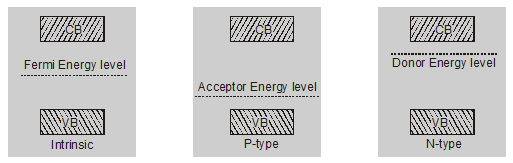Most Affordable JEE | NEET | 8,9,10 Preparation by Kota's Top IITian Doctor Faculties

# What is Fermi energy level in semiconductors - Electronics - eSaral`

Hey, Do you want to know what is fermi energy level in semiconductors? If yes. Then keep reading.

## Fermi Energy Level.

Fermi energy is the maximum kinetic energy of an electron at 0 K these electrons are called Fermi electrons and energy level is known as Fermi energy level.

• It is always found between the conduction band and valance band

• Fermi Level is the energy that corresponds to the center of gravity of the conduction electrons and holes weighted according to their energies.• In pure germanium semiconductor, the Fermi level is about halfway in the forbidden gap.

• In an n-type semiconductor, the Fermi level lies in the forbidden gap, very close to the conduction band.

• In p-type semiconductor, the Fermi level lies in the forbidden gap, very close to the valence band.

• With rising in temperature the Fermi level moves towards the center of the forbidden gap, for both types of semiconductors.

• An n-type semiconductor is better than a p-type semiconductor as electrons have more mobility than holes.

## Mass Action Law

Under thermal equilibrium, the product of concentration $\mathrm{n}_{\mathrm{e}}$ of free electrons and the concentration $\mathbf{n}_{\mathrm{h}}$ of holes is constant & independent of the amount of doping by donor & acceptor impurity.

$\mathrm{n}_{e} \mathrm{n}_{\mathrm{h}}=\mathbf{n}_{\mathrm{i}}^{2}$

where

$\mathrm{n}_{\mathrm{i}}=$ intrinsic concentration

However, the intrinsic concentration is a function of temperature.

• In n-type semiconductors, the number density of electrons is nearly equal to the number density of donor atoms $\mathrm{N}_{\mathrm{d}}$ and is very large as compared to the number density of holes.

$\mathrm{n}_{\mathrm{e}} \approx \mathrm{N}_{\mathrm{D}}$

$N_{D}>>n_{h}$

• In p-type semiconductor, the number density of holes is nearly equal to the number density of acceptor atoms $\mathrm{N}_{\mathrm{a}}$ and is very large as compared to number density of electrons.

$\mathbf{n}_{\mathrm{h}} \approx \mathrm{N}_{\mathrm{A}}$

$\mathrm{N}_{\mathrm{A}}>>\mathrm{n}_{\mathrm{e}}$

So, that's all from this article. I hope you get the idea about what is fermi energy level in semiconductors. If you found this article informative then please share it with your friends. If you have any confusion related to this topic, then you can ask in the comments section down below.

For a better understanding of this chapter, please check the detailed notes of Electronics. To watch Free Learning Videos on physics by Saransh Gupta sir Install the eSaral App.